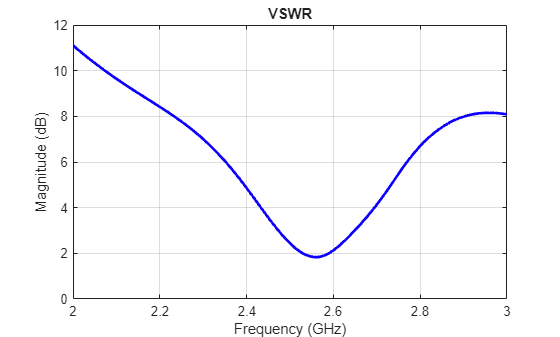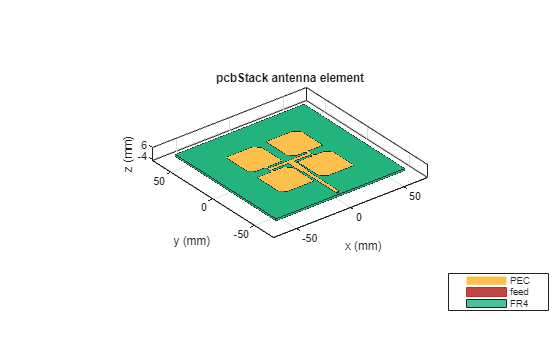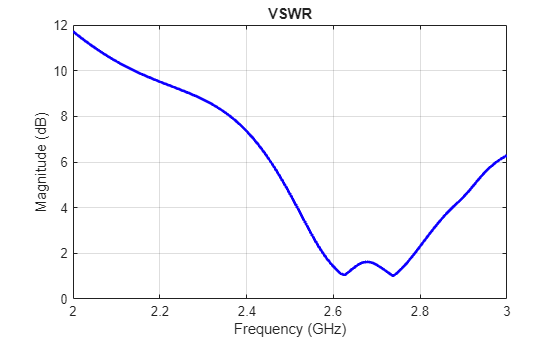# Design, Analyze, and Prototype 2x2 Patch Array Antenna

This example shows how to create a 2x2 patch array on FR4 substrate, analyze the antenna, and generate Gerber files of the PCB for prototyping. The design operates at around 2.4 GHz.

### Design Parameters

Set up the dielectric according to  and specify the physical constants.

```freq = 2.4e9; freqRange = linspace(2e9,3e9); c = physconst("lightspeed"); d = dielectric("FR4"); d.EpsilonR = 4.3; d.Thickness = 1.6e-3;```

### Calculate Patch Dimensions

Find the dimensions of the patch microstrip using the equations in . The width and length are based on the effective permittivity ${ϵ}_{R}$ and effective wavelenth ${\lambda }_{eff}$ in the substrate.

```W = c/(2*freq*sqrt((d.EpsilonR+1)/2)); epsilonEff = (d.EpsilonR+1)/2 + (d.EpsilonR-1)/2/sqrt(1+12*d.Thickness/W); lambdaEff = c/(freq*sqrt(epsilonEff)); Leff = lambdaEff/2; deltaL = 0.412*d.Thickness*(epsilonEff+0.3)*(W/d.Thickness+0.264)/(epsilonEff-0.258)/(W/d.Thickness+0.8); L = Leff - 2*deltaL;```

### Create Patch

Create a square patch microstrip antenna element using the ground plane length and width described in .

```GroundPlaneLength = 0.12; GroundPlaneWidth = 0.12; patch = patchMicrostrip(Substrate=d, Height=d.Thickness, Length=L, Width=L,... GroundPlaneLength=GroundPlaneLength/2, GroundPlaneWidth=GroundPlaneWidth/2,... FeedOffset=[0,0])```
```patch = patchMicrostrip with properties: Length: 0.0298 Width: 0.0298 Height: 0.0016 Substrate: [1×1 dielectric] GroundPlaneLength: 0.0600 GroundPlaneWidth: 0.0600 PatchCenterOffset: [0 0] FeedOffset: [0 0] Conductor: [1×1 metal] Tilt: 0 TiltAxis: [1 0 0] Load: [1×1 lumpedElement] ```

### Create Array

Set the spacing between the elements in the array to be greater than half of the effective wavelength and create the rectangular array.

```spacing = lambdaEff*0.6; arr = rectangularArray(Element=patch, RowSpacing=spacing, ColumnSpacing=spacing)```
```arr = rectangularArray with properties: Element: [1×1 patchMicrostrip] Size: [2 2] RowSpacing: 0.0375 ColumnSpacing: 0.0375 Lattice: 'Rectangular' AmplitudeTaper: 1 PhaseShift: 0 Tilt: 0 TiltAxis: [1 0 0] ```
`arr.Element`
```ans = patchMicrostrip with properties: Length: 0.0298 Width: 0.0298 Height: 0.0016 Substrate: [1×1 dielectric] GroundPlaneLength: 0.0600 GroundPlaneWidth: 0.0600 PatchCenterOffset: [0 0] FeedOffset: [0 0] Conductor: [1×1 metal] Tilt: 0 TiltAxis: [1 0 0] Load: [1×1 lumpedElement] ```

Display the layout and show the array without the traces. You can change this configuration so the bottom of the PCB has only one feedpoint.

`layout(arr)`
```ans=1×2 cell array {1×1 Patch} {1×1 Patch} ``````figure show(arr)```### Create Feed Trace

Create the trace from . The authors use T-junction Wilkinson power dividers to impose a resistance of 50 $\Omega$ at the feedpoints and at the edge of the patches. This figure from paper  shows the impedances of the traces.```z0 = 50; traceWidth = traceThickness(z0,d)```
```traceWidth = 0.0031 ```
```z1 = real(z0)*sqrt(2); traceWidth2 = traceThickness(z1,d)```
```traceWidth2 = 0.0017 ```
```z2 = real(z0)/sqrt(2); traceWidth3 = traceThickness(z2,d)```
```traceWidth3 = 0.0053 ```
```offset = 3e-3; feedLocation = -arr.GroundPlaneWidth/2 + offset; x = abs(arr.FeedLocation(1,1)); y = abs(arr.FeedLocation(1,2)); firstTLength = 2*y; secondTLength = 2*x + traceWidth2; feedLength = traceWidth/2 - feedLocation; feedTraceCenter = feedLocation + feedLength/2; yLower = y - L/2; patchFeedLength = L/2 + yLower/4; patchFeedCenter = y - patchFeedLength/2; feedTLength = feedLength/3; feedTCenter = traceWidth/2 - feedTLength/2; t1 = antenna.Rectangle(Length=traceWidth2, Width=firstTLength, Center=[x 0]); t2 = antenna.Rectangle(Length=traceWidth2, Width=firstTLength, Center=[-x 0]); t3 = antenna.Rectangle(Length=secondTLength, Width=traceWidth, Center=[0 0]); t4 = antenna.Rectangle(Length=traceWidth, Width=feedLength, Center=[0 feedTraceCenter]); t5 = antenna.Rectangle(Length=traceWidth, Width=patchFeedLength, Center=[x patchFeedCenter]); t6 = antenna.Rectangle(Length=traceWidth, Width=patchFeedLength, Center=[-x patchFeedCenter]); t7 = antenna.Rectangle(Length=traceWidth, Width=patchFeedLength, Center=[x -patchFeedCenter]); t8 = antenna.Rectangle(Length=traceWidth, Width=patchFeedLength, Center=[-x -patchFeedCenter]); t9 = antenna.Rectangle(Length=traceWidth3, Width=feedTLength, Center=[0 feedTCenter]); feedTrace = t1 + t2 + t3 + t4 + t5 + t6 + t7 + t8 + t9; figure(Name="Feed Trace") show(feedTrace)```### Sweep Side Lengths of Corners

Determine how much to cut off the corners by sweeping through possible side lengths of the triangle to cut and determine the impedance $z$ of the PCB. $S$ represents the ratio of triangle side length to the patch's length, so $S$ is the side length of the triangle that must be cut from the patch. Truncating the corners helps match the impedance to the desired value of 50 $\Omega$ and the resonant frequency to 2.4 GHz.

The `impedanceSweep` MAT file contains a saved value of the impedance $z$ of a PCB with truncated corners whose side lengths are equal to $S\cdot L$. Because computing the impedances for all the differerent values of $S$ takes several hours, using the stored value in the MAT file speeds up the example. To calculate the value of `z` using the `sweepImpedance` function, set the `calculateImpedance` variable to `true`. The sweepImpedance function loops through values of $S$ to generate a PCB with truncated corners whose side lengths correspond to those values.

Plot the real and imaginary imaginary impedance as functions of $S$.

```S = linspace(0.15,0.165,500); calculateImpedance = false; if calculateImpedance z = sweepImpedance(arr,S); else load("impedanceSweep.mat"); end figure plot(S,real(z)) hold on plot(S,imag(z)) hold off```### Create Truncated Corners

Find the value of $S$ that results in the PCB having impedance values closest to 50 $\Omega$ resistance and 0 $\Omega$ reactance. For this design, cut off isosceles right triangles with leg lengths equal to roughly 16% of the side length of a patch, or 4.7 mm.

`[zmin,zidx] = min(abs(z-z0))`
```zmin = 2.5609 ```
```zidx = 318 ```
`z(zidx)`
```ans = 47.6976 + 1.1212i ```
`S(zidx)`
```ans = 0.1595 ```
`s = S(zidx)*L`
```s = 0.0047 ```
```truncatedCorners = createTruncatedCorners(arr,s); show(truncatedCorners)```### Create PCB Stack Without Truncated Corners

Convert the array to a PCB stack and join the feed trace with the array. Then, set the feed and via locations and properties. Ensure that the PCB dielectric is as big as the groundplane.

```arrPCB = pcbStack(arr); arrPCB.Layers{1,1} = arrPCB.Layers{1,1} + feedTrace; arrPCB.FeedLocations = [0 feedLocation 1 3]; arrPCB.ViaLocations = arrPCB.FeedLocations(1,:); arrPCB.FeedDiameter = traceWidth/2; arrPCB.ViaDiameter = arrPCB.FeedDiameter; arrPCB.Layers{1,2}.Length = GroundPlaneLength; arrPCB.Layers{1,2}.Width = GroundPlaneWidth; figure; show(arrPCB);```### Analyze PCB Stack Without Truncated Corners

Analyze the PCB stack of the array that does not have truncated corners. Determine the return loss, the voltage standing wave ratio, the impedance, and the S-parameters.

`returnLoss(arrPCB,freqRange,z0);``vswr(arrPCB,freqRange,z0);``impedance(arrPCB,freqRange);````spar = sparameters(arrPCB,freqRange,z0); rfplot(spar)```Show the PCB radiation pattern at 2.4 GHz.

`pattern(arrPCB,freq)`### Create PCB Stack with Truncated Corners

Convert the array to a PCB stack and join the feed trace with the array. Truncate the corners from the patches. Then, set the feed and via locations and properties. Ensure that the PCB's dielectric is as big as the groundplane.

```arrPCB_tc = pcbStack(arr); arrPCB_tc.Layers{1,1} = arrPCB_tc.Layers{1,1} + feedTrace - truncatedCorners; arrPCB_tc.FeedLocations = [0 feedLocation 1 3]; arrPCB_tc.ViaLocations = arrPCB_tc.FeedLocations(1,:); arrPCB_tc.FeedDiameter = traceWidth/2; arrPCB_tc.ViaDiameter = arrPCB_tc.FeedDiameter; arrPCB_tc.Layers{1,2}.Length = GroundPlaneLength; arrPCB_tc.Layers{1,2}.Width = GroundPlaneWidth; figure; show(arrPCB_tc);```### Analyze PCB Stack with Truncated Corners

Analyze the PCB Stack of the array with truncated corners. Determine the return loss, the voltage standing wave ratio, the impedance, and the S-parameters.

`returnLoss(arrPCB_tc,freqRange,z0);``vswr(arrPCB_tc,freqRange,z0);``impedance(arrPCB_tc,freqRange);````spar = sparameters(arrPCB_tc,freqRange,z0); rfplot(spar)```Show the PCB radiation pattern at 2.4 GHz.

`pattern(arrPCB_tc,freq)`### Generate Gerber Files

You can use Gerber files to export the geometry information of a PCB. Use a PCB writer and an RF connector to generate the Gerber files.

```W = PCBServices.OSHParkWriter; W = PCBServices.MayhewWriter; W.Filename = 'patch array 2x2'; C = SMAEdge_SamtecCustom; C.EdgeLocation = "south"; C.ExtendBoardProfile = false; Am = PCBWriter(arrPCB_tc,W,C); gerberWrite(Am)```

If you have an internet connection, a browser window opens and displays the Mayhewlabs free 3D online Gerber viewer. After you drag and drop the files into the Mayhewslabs viewer, the software renders the PCB design. This figure shows the front view of the board.This figure shows the back view of the board.### Conclusion

In this example, you create a 2x2 patch array on FR4, analyze the antenna, and generate Gerber files of the PCB for prototyping.

Without truncated corners, the array has a single resonant frequency at 2.38 GHz. The reactance reaches 0 $\Omega$ at around 2.45 GHz.

With the truncated corners, the array as multiple frequencies with ${S}_{11}\le -10$ dB in the S-parameter plot, one minimum at 2.39 GHz, and another minimum at 2.48 GHz. The reactance reaches 0 $\Omega$ at around 2.41 GHz.

### Supporting Functions

#### Determine Trace Widths

The `traceThickness` function determines the thickness of the traces on the PCB for input impedance and dielectric based on the equation from .

```function width = traceThickness(z,d) A = z/60*sqrt((d.EpsilonR+1)/2)+(d.EpsilonR-1)/(d.EpsilonR+1)*(0.23+0.11/d.EpsilonR); width = d.Thickness*8*exp(A)/(exp(2*A)-2); end```

#### Create the Truncated Corners

The `truncatedCorners` function creates the truncated corners for the input array and leg length of an isosceles right triangle.

```function truncatedCorners = createTruncatedCorners(arr,s) x11 = -arr.ColumnSpacing/2 + arr.Element.Length/2; y11 = arr.RowSpacing/2 + arr.Element.Width/2; x12 = -arr.ColumnSpacing/2 - arr.Element.Length/2; y12 = arr.RowSpacing/2 - arr.Element.Width/2; s1 = antenna.Polygon(Vertices=[x11-s y11 0; x11 y11-s 0; x11 y11 0]); s2 = antenna.Polygon(Vertices=[x12+s y12 0; x12 y12+s 0; x12 y12 0]); x21 = x11; y21 = -arr.RowSpacing/2+arr.Element.Width/2; x22 = x12; y22 = -arr.RowSpacing/2-arr.Element.Width/2; s3 = antenna.Polygon(Vertices=[x21-s y21 0; x21 y21-s 0; x21 y21 0]); s4 = antenna.Polygon(Vertices=[x22+s y22 0; x22 y22+s 0; x22 y22 0]); x31 = arr.ColumnSpacing/2 + arr.Element.Length/2; y31 = y11; x32 = arr.ColumnSpacing/2 - arr.Element.Length/2; y32 = y12; s5 = antenna.Polygon(Vertices=[x31-s y31 0; x31 y31-s 0; x31 y31 0]); s6 = antenna.Polygon(Vertices=[x32+s y32 0; x32 y32+s 0; x32 y32 0]); x41 = x31; y41 = y21; x42 = x32; y42 = y22; s7 = antenna.Polygon(Vertices=[x41-s y41 0; x41 y41-s 0; x41 y41 0]); s8 = antenna.Polygon(Vertices=[x42+s y42 0; x42 y42+s 0; x42 y42 0]); truncatedCorners = s1 + s2 + s3 + s4 + s5 + s6 + s7 + s8; end```

#### Calculate Impedance

For each element of the ratio `S` of truncted corner side length to patch length, the `sweepImpedance` function creates the truncated corners. The function then creates the PCB stack of the array, adds the feed trace, and removes the corners. You specify the feed and via locations and properties, ensuring that the PCB's dielectric is as big as the groundplane. Finally, the function computes the impedance of the PCB.

```function z = sweepImpedance(arr,S) z = zeros(size(S)); for i = 1:length(S) s = S(i)*L; truncatedCorners = createTruncatedCorners(arr,s); pcb = pcbStack(arr); pcb.Layers{1,1} = pcb.Layers{1,1} + feedTrace - truncatedCorners; pcb.FeedLocations = [0 feedLocation 1 3]; pcb.ViaLocations = pcb.FeedLocations(1,:); pcb.FeedDiameter = traceWidth/2; pcb.ViaDiameter = pcb.FeedDiameter; pcb.Layers{1,2}.Length = GroundPlaneLength; pcb.Layers{1,2}.Width = GroundPlaneWidth; z(i) = impedance(pcb,freq); end end```

### Reference

 Muludi, Zainal, and Budi Aswoyo. “Truncated Microstrip Square Patch Array Antenna 2 × 2 Elements with Circular Polarization for S-Band Microwave Frequency.” In 2017 International Electronics Symposium on Engineering Technology and Applications (IES-ETA), 87–92. Surabaya: IEEE, 2017. https://doi.org/10.1109/ELECSYM.2017.8240384.

 Balanis, Constantine A. Antenna Theory: Analysis and Design. Fourth edition. Hoboken, New Jersey: Wiley, 2016.